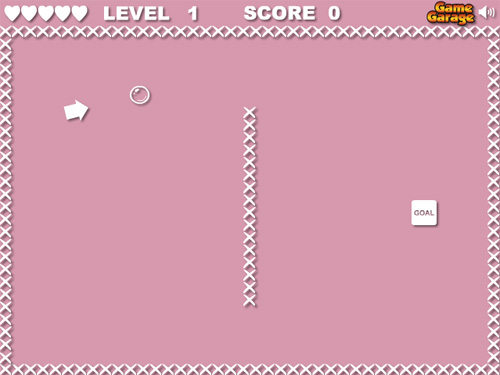# Prototype of a Flash game like Floaty Light

Do we really need another “move a ball in some way from A to B avoiding C” game?Noooooooo… but it seems two or three people still like this kind of games, so the new torture branded Game Garage is Floaty Light.

Just kidding, of course.

I made this prototype using the gravity and speed as described in the Flash game creation tutorial – part 1 post, the collision detection as shown in the Create a flash draw game like Line Rider or others – part 2 post and the control system already seen at A new player control concept, with only a minor change.

As said, nothing new, but it’s an interesting concept anyway

```_root.attachMovie("ball", "ball", _root.getNextHighestDepth(), {_x:50, _y:50});
_root.attachMovie("wall", "wall", _root.getNextHighestDepth(), {_x:240, _y:200});
_root.attachMovie("arrow", "arrow", _root.getNextHighestDepth());
moving = false;
gravity = 0.01;
xspeed = 0;
yspeed = 0;
precision = 24;
Mouse.hide();
arrow.onEnterFrame = function() {
this._x = _xmouse;
this._y = _ymouse;
dist_x = ball._x-this._x;
dist_y = ball._y-this._y;
total_dist = Math.sqrt(dist_x*dist_x+dist_y*dist_y);
if (total_dist>300) {
total_dist = 300;
}
total_dist = (300-total_dist)/500;
angle = Math.atan2(dist_y, dist_x);
this._rotation = angle*57.2957795;
};
ball.onEnterFrame = function() {
if (moving) {
dir = arrow._rotation;
xspeed += total_dist*Math.cos(dir*0.0174532925);
yspeed += total_dist*Math.sin(dir*0.0174532925);
}
yspeed += gravity;
this._x += xspeed;
this._y += yspeed;
xspeed *= 0.99;
yspeed *= 0.99;
for (x=1; x
Let's play it!

```
214 GAME PROTOTYPES EXPLAINED WITH SOURCE CODE
// 1+2=3
// 10000000
// 2 Cars
// 2048
// Avoider
// Ballz
// Block it
// Blockage
// Bloons
// Boids
// Bombuzal
// Breakout
// Bricks
// Columns
// CubesOut
// Dots
// DROP'd
// Dudeski
// Eskiv
// Filler
// Fling
// Globe
// HookPod
// Hundreds
// InkTd
// Iromeku
// Lumines
// Magick
// MagOrMin
// Maze
// Memdot
// Nano War
// Nodes
// o:anquan
// Ononmin
// Pacco
// Phyballs
// Platform
// Poker
// Pool
// Poux
// Pudi
// qomp
// Racing
// Renju
// SameGame
// Security
// Sling
// Slingy
// Sokoban
// Splitter
// Sproing
// Stack
// Stringy
// Sudoku
// Tetris
// Threes
// Toony
// Turn
// TwinSpin
// vvvvvv
// Wordle
// Worms
// Yanga
// Zhed
// zNumbers